# Example Codes and Miniapps

This page provides a brief overview of MFEM's example codes and miniapps. For detailed documentation of the MFEM sources, including the examples, see the online Doxygen documentation, or the doc directory in the distribution.

The goal of the example codes is to provide a step-by-step introduction to MFEM in simple model settings. The miniapps are more complex, and are intended to be more representative of the advanced usage of the library in physics/application codes. We recommend that new users start with the example codes before moving to the miniapps.

Clicking on any of the categories below displays examples and miniapps that contain the described feature. All examples support (arbitrarily) high-order meshes and finite element spaces. The numerical results from the example codes can be visualized using the GLVis visualization tool (based on MFEM). See the GLVis website for more details.

Users are encouraged to submit any example codes and miniapps that they have created and would like to share.
Contact a member of the MFEM team to report bugs or post questions or comments.

## Example 1: Laplace Problem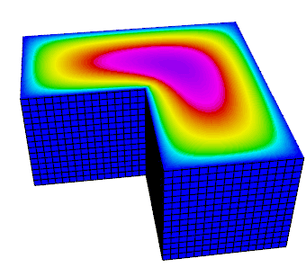This example code demonstrates the use of MFEM to define a simple isoparametric finite element discretization of the Laplace problem $$-\Delta u = 1$$ with homogeneous Dirichlet boundary conditions. Specifically, we discretize with the finite element space coming from the mesh (linear by default, quadratic for quadratic curvilinear mesh, NURBS for NURBS mesh, etc.)

The example highlights the use of mesh refinement, finite element grid functions, as well as linear and bilinear forms corresponding to the left-hand side and right-hand side of the discrete linear system. We also cover the explicit elimination of essential boundary conditions, static condensation, and the optional connection to the GLVis tool for visualization.

The example has a serial (ex1.cpp), a parallel (ex1p.cpp), and HPC versions: performance/ex1.cpp, performance/ex1p.cpp. It also has a PETSc modification in examples/petsc and a PUMI modification in examples/pumi.

## Example 2: Linear Elasticity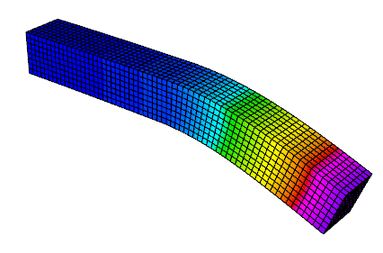This example code solves a simple linear elasticity problem describing a multi-material cantilever beam. Specifically, we approximate the weak form of $$-{\rm div}({\sigma}({\bf u})) = 0$$ where $${\sigma}({\bf u}) = \lambda\, {\rm div}({\bf u})\,I + \mu\,(\nabla{\bf u} + \nabla{\bf u}^T)$$ is the stress tensor corresponding to displacement field ${\bf u}$, and $\lambda$ and $\mu$ are the material Lame constants. The boundary conditions are ${\bf u}=0$ on the fixed part of the boundary with attribute 1, and ${\sigma}({\bf u})\cdot n = f$ on the remainder with $f$ being a constant pull down vector on boundary elements with attribute 2, and zero otherwise. The geometry of the domain is assumed to be as follows: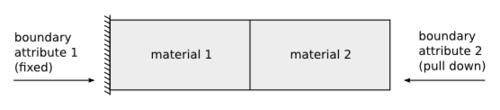The example demonstrates the use of high-order and NURBS vector finite element spaces with the linear elasticity bilinear form, meshes with curved elements, and the definition of piece-wise constant and vector coefficient objects. Static condensation is also illustrated.

The example has a serial (ex2.cpp) and a parallel (ex2p.cpp) version. It also has a PETSc modification in examples/petsc and a PUMI modification in examples/pumi. We recommend viewing Example 1 before viewing this example.

## Example 3: Definite Maxwell Problem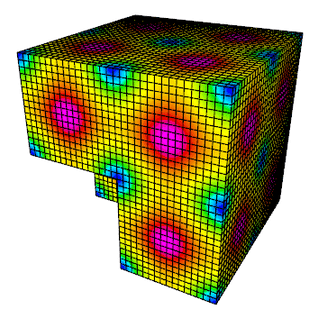This example code solves a simple 3D electromagnetic diffusion problem corresponding to the second order definite Maxwell equation $$\nabla\times\nabla\times\, E + E = f$$ with boundary condition $E \times n$ = "given tangential field". Here, we use a given exact solution $E$ and compute the corresponding r.h.s. $f$. We discretize with Nedelec finite elements in 2D or 3D.

The example demonstrates the use of $H(curl)$ finite element spaces with the curl-curl and the (vector finite element) mass bilinear form, as well as the computation of discretization error when the exact solution is known. Static condensation is also illustrated.

The example has a serial (ex3.cpp) and a parallel (ex3p.cpp) version. It also has a PETSc modification in examples/petsc. We recommend viewing examples 1-2 before viewing this example.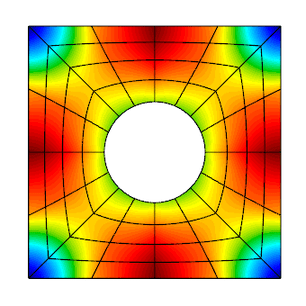This example code solves a simple 2D/3D $H(div)$ diffusion problem corresponding to the second order definite equation $$-{\rm grad}(\alpha\,{\rm div}(F)) + \beta F = f$$ with boundary condition $F \cdot n$ = "given normal field". Here we use a given exact solution $F$ and compute the corresponding right hand side $f$. We discretize with the Raviart-Thomas finite elements.

The example demonstrates the use of $H(div)$ finite element spaces with the grad-div and $H(div)$ vector finite element mass bilinear form, as well as the computation of discretization error when the exact solution is known. Bilinear form hybridization and static condensation are also illustrated.

The example has a serial (ex4.cpp) and a parallel (ex4p.cpp) version. It also has a PETSc modification in examples/petsc. We recommend viewing examples 1-3 before viewing this example.

## Example 5: Darcy Problem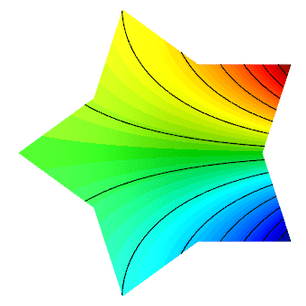This example code solves a simple 2D/3D mixed Darcy problem corresponding to the saddle point system $$\begin{array}{rcl} k\,{\bf u} + {\rm grad}\,p &=& f \\ -{\rm div}\,{\bf u} &=& g \end{array}$$ with natural boundary condition $-p =$ "given pressure". Here we use a given exact solution $({\bf u},p)$ and compute the corresponding right hand side $(f, g)$. We discretize with Raviart-Thomas finite elements (velocity $\bf u$) and piecewise discontinuous polynomials (pressure $p$).

The example demonstrates the use of the BlockMatrix and BlockOperator classes, as well as the collective saving of several grid functions in a VisIt visualization format.

The example has a serial (ex5.cpp) and a parallel (ex5p.cpp) version. It also has a PETSc modification in examples/petsc. We recommend viewing examples 1-4 before viewing this example.

## Example 6: Laplace Problem with AMR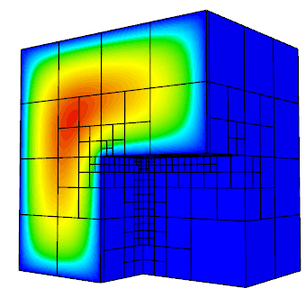This is a version of Example 1 with a simple adaptive mesh refinement loop. The problem being solved is again the Laplace equation $$-\Delta u = 1$$ with homogeneous Dirichlet boundary conditions. The problem is solved on a sequence of meshes which are locally refined in a conforming (triangles, tetrahedrons) or non-conforming (quadrilaterals, hexahedra) manner according to a simple ZZ error estimator.

The example demonstrates MFEM's capability to work with both conforming and nonconforming refinements, in 2D and 3D, on linear, curved and surface meshes. Interpolation of functions from coarse to fine meshes, as well as persistent GLVis visualization are also illustrated.

The example has a serial (ex6.cpp) and a parallel (ex6p.cpp) version. It also has a PETSc modification in examples/petsc and a PUMI modification in examples/pumi. We recommend viewing Example 1 before viewing this example.

## Example 7: Surface Meshes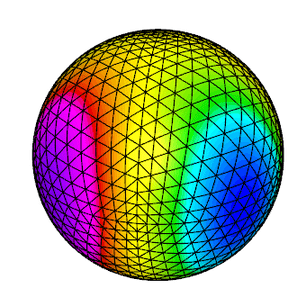This example code demonstrates the use of MFEM to define a triangulation of a unit sphere and a simple isoparametric finite element discretization of the Laplace problem with mass term, $$-\Delta u + u = f.$$

The example highlights mesh generation, the use of mesh refinement, high-order meshes and finite elements, as well as surface-based linear and bilinear forms corresponding to the left-hand side and right-hand side of the discrete linear system. Simple local mesh refinement is also demonstrated.

The example has a serial (ex7.cpp) and a parallel (ex7p.cpp) version. We recommend viewing Example 1 before viewing this example.

## Example 8: DPG for the Laplace Problem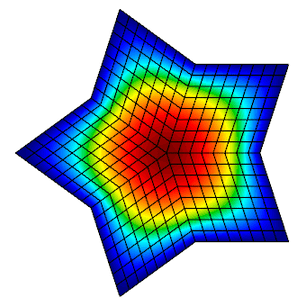This example code demonstrates the use of the Discontinuous Petrov-Galerkin (DPG) method in its primal 2x2 block form as a simple finite element discretization of the Laplace problem $$-\Delta u = f$$ with homogeneous Dirichlet boundary conditions. We use high-order continuous trial space, a high-order interfacial (trace) space, and a high-order discontinuous test space defining a local dual ($H^{-1}$) norm. We use the primal form of DPG, see "A primal DPG method without a first-order reformulation", Demkowicz and Gopalakrishnan, CAM 2013.

The example highlights the use of interfacial (trace) finite elements and spaces, trace face integrators and the definition of block operators and preconditioners.

The example has a serial (ex8.cpp) and a parallel (ex8p.cpp) version. We recommend viewing examples 1-5 before viewing this example.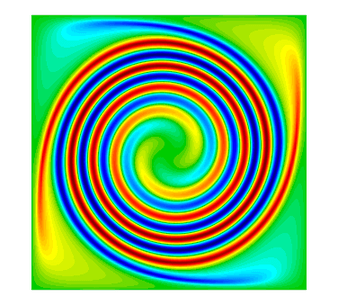This example code solves the time-dependent advection equation $$\frac{\partial u}{\partial t} + v \cdot \nabla u = 0,$$ where $v$ is a given fluid velocity, and $u_0(x)=u(0,x)$ is a given initial condition.

The example demonstrates the use of Discontinuous Galerkin (DG) bilinear forms in MFEM (face integrators), the use of explicit ODE time integrators, the definition of periodic boundary conditions through periodic meshes, as well as the use of GLVis for persistent visualization of a time-evolving solution. The saving of time-dependent data files for external visualization with VisIt is also illustrated.

The example has a serial (ex9.cpp) and a parallel (ex9p.cpp) version. It also has a SUNDIALS modification in examples/sundials and a PETSc modification in examples/petsc.

## Example 10: Nonlinear Elasticity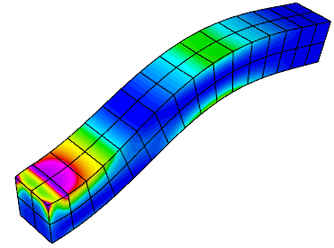This example solves a time dependent nonlinear elasticity problem of the form $$\frac{dv}{dt} = H(x) + S v\,,\qquad \frac{dx}{dt} = v\,,$$ where $H$ is a hyperelastic model and $S$ is a viscosity operator of Laplacian type. The geometry of the domain is assumed to be as follows: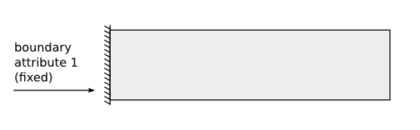The example demonstrates the use of nonlinear operators, as well as their implicit time integration using a Newton method for solving an associated reduced backward-Euler type nonlinear equation. Each Newton step requires the inversion of a Jacobian matrix, which is done through a (preconditioned) inner solver.

The example has a serial (ex10.cpp) and a parallel (ex10p.cpp) version. It also has a SUNDIALS modification in examples/sundials and a PETSc modification in examples/petsc. We recommend viewing examples 2 and 9 before viewing this example.

## Example 11: Laplace Eigenproblem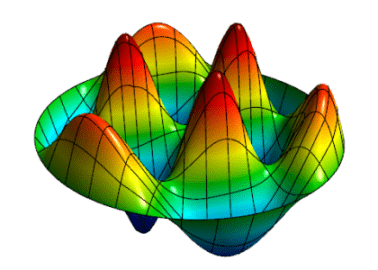This example code demonstrates the use of MFEM to solve the eigenvalue problem $$-\Delta u = \lambda u$$ with homogeneous Dirichlet boundary conditions.

We compute a number of the lowest eigenmodes by discretizing the Laplacian and Mass operators using a finite element space of the specified order, or an isoparametric/isogeometric space if order < 1 (quadratic for quadratic curvilinear mesh, NURBS for NURBS mesh, etc.)

The example highlights the use of the LOBPCG eigenvalue solver together with the BoomerAMG preconditioner in HYPRE, as well as optionally the SuperLU or STRUMPACK parallel direct solvers. Reusing a single GLVis visualization window for multiple eigenfunctions is also illustrated.

The example has only a parallel (ex11p.cpp) version. We recommend viewing Example 1 before viewing this example.

## Example 12: Linear Elasticity Eigenproblem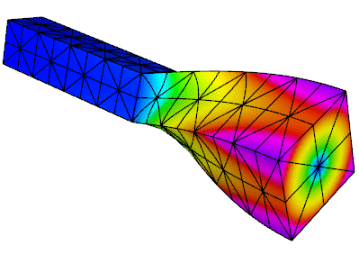This example code solves the linear elasticity eigenvalue problem for a multi-material cantilever beam. Specifically, we compute a number of the lowest eigenmodes by approximating the weak form of $$-{\rm div}({\sigma}({\bf u})) = \lambda {\bf u} \,,$$ where $${\sigma}({\bf u}) = \lambda\, {\rm div}({\bf u})\,I + \mu\,(\nabla{\bf u} + \nabla{\bf u}^T)$$ is the stress tensor corresponding to displacement field $\bf u$, and $\lambda$ and $\mu$ are the material Lame constants. The boundary conditions are ${\bf u}=0$ on the fixed part of the boundary with attribute 1, and ${\sigma}({\bf u})\cdot n = f$ on the remainder. The geometry of the domain is assumed to be as follows: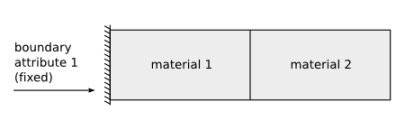The example highlights the use of the LOBPCG eigenvalue solver together with the BoomerAMG preconditioner in HYPRE. Reusing a single GLVis visualization window for multiple eigenfunctions is also illustrated.

The example has only a parallel (ex12p.cpp) version. We recommend viewing examples 2 and 11 before viewing this example.

## Example 13: Maxwell Eigenproblem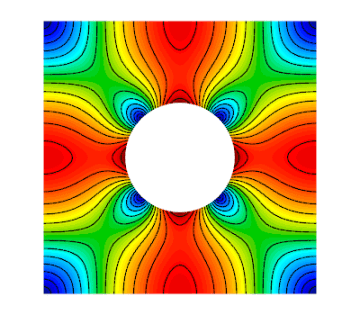This example code solves the Maxwell (electromagnetic) eigenvalue problem $$\nabla\times\nabla\times\, E = \lambda\, E$$ with homogeneous Dirichlet boundary conditions $E \times n = 0$.

We compute a number of the lowest nonzero eigenmodes by discretizing the curl curl operator using a Nedelec finite element space of the specified order in 2D or 3D.

The example highlights the use of the AME subspace eigenvalue solver from HYPRE, which uses LOBPCG and AMS internally. Reusing a single GLVis visualization window for multiple eigenfunctions is also illustrated.

The example has only a parallel (ex13p.cpp) version. We recommend viewing examples 3 and 11 before viewing this example.

## Example 14: DG Diffusion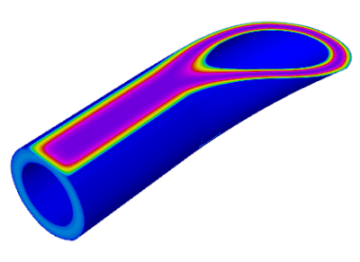This example code demonstrates the use of MFEM to define a discontinuous Galerkin (DG) finite element discretization of the Laplace problem $$-\Delta u = 1$$ with homogeneous Dirichlet boundary conditions. Finite element spaces of any order, including zero on regular grids, are supported. The example highlights the use of discontinuous spaces and DG-specific face integrators.

The example has a serial (ex14.cpp) and a parallel (ex14p.cpp) version. We recommend viewing examples 1 and 9 before viewing this example.

## Example 15: Dynamic AMR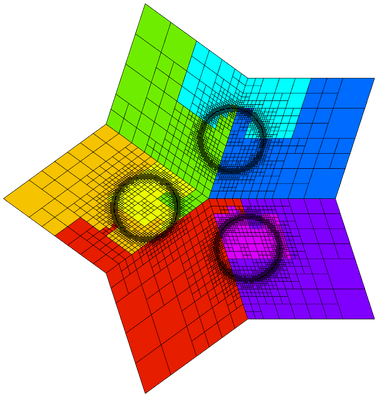Building on Example 6, this example demonstrates dynamic adaptive mesh refinement. The mesh is adapted to a time-dependent solution by refinement as well as by derefinement. For simplicity, the solution is prescribed and no time integration is done. However, the error estimation and refinement/derefinement decisions are realistic.

At each outer iteration the right hand side function is changed to mimic a time dependent problem. Within each inner iteration the problem is solved on a sequence of meshes which are locally refined according to a simple ZZ error estimator. At the end of the inner iteration the error estimates are also used to identify any elements which may be over-refined and a single derefinement step is performed. After each refinement or derefinement step a rebalance operation is performed to keep the mesh evenly distributed among the available processors.

The example demonstrates MFEM's capability to refine, derefine and load balance nonconforming meshes, in 2D and 3D, and on linear, curved and surface meshes. Interpolation of functions between coarse and fine meshes, persistent GLVis visualization, and saving of time-dependent fields for external visualization with VisIt are also illustrated.

The example has a serial (ex15.cpp) and a parallel (ex15p.cpp) version. We recommend viewing examples 1, 6 and 9 before viewing this example.

## Example 16: Time Dependent Heat Conduction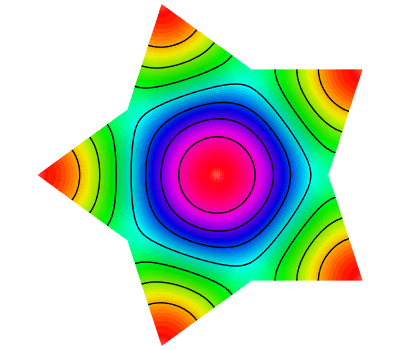This example code solves a simple 2D/3D time dependent nonlinear heat conduction problem $$\frac{du}{dt} = \nabla \cdot \left( \kappa + \alpha u \right) \nabla u$$ with a natural insulating boundary condition $\frac{du}{dn} = 0$. We linearize the problem by using the temperature field $u$ from the previous time step to compute the conductivity coefficient.

This example demonstrates both implicit and explicit time integration as well as a single Picard step method for linearization. The saving of time dependent data files for external visualization with VisIt is also illustrated.

The example has a serial (ex16.cpp) and a parallel (ex16p.cpp) version. We recommend viewing examples 2, 9, and 10 before viewing this example.

## Example 17: DG Linear Elasticity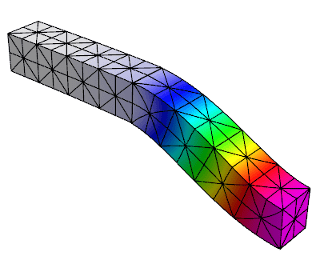This example code solves a simple linear elasticity problem describing a multi-material cantilever beam using symmetric or non-symmetric discontinuous Galerkin (DG) formulation.

Specifically, we approximate the weak form of $$-{\rm div}({\sigma}({\bf u})) = 0$$ where $${\sigma}({\bf u}) = \lambda\, {\rm div}({\bf u})\,I + \mu\,(\nabla{\bf u} + \nabla{\bf u}^T)$$ is the stress tensor corresponding to displacement field ${\bf u}$, and $\lambda$ and $\mu$ are the material Lame constants. The boundary conditions are Dirichlet, $\bf{u}=\bf{u_D}$, on the fixed part of the boundary, namely boundary attributes 1 and 2; on the rest of the boundary we use ${\sigma}({\bf u})\cdot n = {\bf 0}$. The geometry of the domain is assumed to be as follows: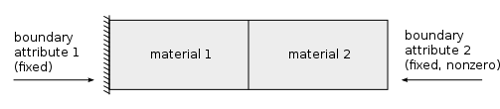The example demonstrates the use of high-order DG vector finite element spaces with the linear DG elasticity bilinear form, meshes with curved elements, and the definition of piece-wise constant and function vector-coefficient objects. The use of non-homogeneous Dirichlet b.c. imposed weakly, is also illustrated.

The example has a serial (ex17.cpp) and a parallel (ex17p.cpp) version. We recommend viewing examples 2 and 14 before viewing this example.

## Example 18: DG Euler Equations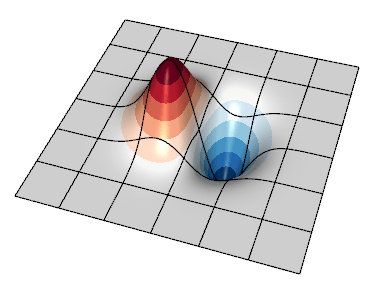This example code solves the compressible Euler system of equations, a model nonlinear hyperbolic PDE, with a discontinuous Galerkin (DG) formulation. The primary purpose is to show how a transient system of nonlinear equations can be formulated in MFEM. The equations are solved in conservative form

$$\frac{\partial u}{\partial t} + \nabla \cdot {\bf F}(u) = 0$$

with a state vector $u = [ \rho, \rho v_0, \rho v_1, \rho E ]$, where $\rho$ is the density, $v_i$ is the velocity in the $i^{\rm th}$ direction, $E$ is the total specific energy, and $H = E + p / \rho$ is the total specific enthalpy. The pressure, $p$ is computed through a simple equation of state (EOS) call. The conservative hydrodynamic flux ${\bf F}$ in each direction $i$ is

$${\bf F_{\it i}} = [ \rho v_i, \rho v_0 v_i + p \delta_{i,0}, \rho v_1 v_i + p \delta_{i,1}, \rho v_i H ]$$

Specifically, the example solves for an exact solution of the equations whereby a vortex is transported by a uniform flow. Since all boundaries are periodic here, the method's accuracy can be assessed by measuring the difference between the solution and the initial condition at a later time when the vortex returns to its initial location.

Note that as the order of the spatial discretization increases, the timestep must become smaller. This example currently uses a simple estimate derived by Cockburn and Shu for the 1D RKDG method. An additional factor can be tuned by passing the --cfl (or -c shorter) flag.

The example demonstrates user-defined bilinear and nonlinear form integrators for systems of equations that are defined with block vectors, and how these are used with an operator for explicit time integrators. In this case the system also involves an external approximate Riemann solver for the DG interface flux. It also demonstrates how to use GLVis for in-situ visualization of vector grid functions.

The example has a serial (ex18.cpp) and a parallel (ex18p.cpp) version. We recommend viewing examples 9, 14 and 17 before viewing this example.

## Example 19: Incompressible Nonlinear Elasticity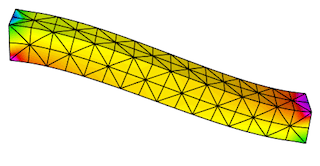This example code solves the quasi-static incompressible nonlinear hyperelasticity equations. Specifically, it solves the nonlinear equation $$\nabla \cdot \sigma(F) = 0$$ subject to the constraint $$\text{det } F = 1$$ where $\sigma$ is the Cauchy stress and $F_{ij} = \delta_{ij} + u_{i,j}$ is the deformation gradient. To handle the incompressibility constraint, pressure is included as an independent unknown $p$ and the stress response is modeled as an incompressible neo-Hookean hyperelastic solid. The geometry of the domain is assumed to be as follows: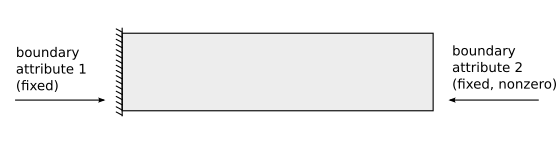This formulation requires solving the saddle point system $$\left[ \begin{array}{cc} K &B^T \\ B & 0 \end{array} \right] \left[\begin{array}{c} \Delta u \\ \Delta p \end{array} \right] = \left[\begin{array}{c} R_u \\ R_p \end{array} \right]$$ at each Newton step. To solve this linear system, we implement a specialized block preconditioner of the form $$P^{-1} = \left[\begin{array}{cc} I & -\tilde{K}^{-1}B^T \\ 0 & I \end{array} \right] \left[\begin{array}{cc} \tilde{K}^{-1} & 0 \\ 0 & -\gamma \tilde{S}^{-1} \end{array} \right]$$ where $\tilde{K}^{-1}$ is an approximation of the inverse of the stiffness matrix $K$ and $\tilde{S}^{-1}$ is an approximation of the inverse of the Schur complement $S = BK^{-1}B^T$. To approximate the Schur complement, we use the mass matrix for the pressure variable $p$.

The example demonstrates how to solve nonlinear systems of equations that are defined with block vectors as well as how to implement specialized block preconditioners for use in iterative solvers.

The example has a serial (ex19.cpp) and a parallel (ex19p.cpp) version. We recommend viewing examples 2, 5 and 10 before viewing this example.

## Example 20: Symplectic Integration of Hamiltonian SystemsThis example demonstrates the use of the variable order, symplectic time integration algorithm. Symplectic integration algorithms are designed to conserve energy when integrating systems of ODEs which are derived from Hamiltonian systems.

Hamiltonian systems define the energy of a system as a function of time (t), a set of generalized coordinates (q), and their corresponding generalized momenta (p). $$H(q,p,t) = T(p) + V(q,t)$$ Hamilton's equations then specify how q and p evolve in time: $$\frac{dq}{dt} = \frac{dH}{dp}\,,\qquad \frac{dp}{dt} = -\frac{dH}{dq}$$

To use the symplectic integration classes we need to define an mfem::Operator ${\bf P}$ which evaluates the action of dH/dp, and an mfem::TimeDependentOperator ${\bf F}$ which computes -dH/dq.

This example visualizes its results as an evolution in phase space by defining the axes to be $q$, $p$, and $t$ rather than $x$, $y$, and $z$. In this space we build a ribbon-like mesh with nodes at $(0,0,t)$ and $(q,p,t)$. Finally we plot the energy as a function of time as a scalar field on this ribbon-like mesh. This scheme highlights any variations in the energy of the system.

This example offers five simple 1D Hamiltonians:

• Simple Harmonic Oscillator (mass on a spring) $$H = \frac{1}{2}\left( \frac{p^2}{m} + \frac{q^2}{k} \right)$$
• Pendulum $$H = \frac{1}{2}\left[ \frac{p^2}{m} - k \left( 1 - cos(q) \right) \right]$$
• Gaussian Potential Well $$H = \frac{p^2}{2m} - k e^{-q^2 / 2}$$
• Quartic Potential $$H = \frac{1}{2}\left[ \frac{p^2}{m} + k \left( 1 + q^2 \right) q^2 \right]$$
• Negative Quartic Potential $$H = \frac{1}{2}\left[ \frac{p^2}{m} + k \left( 1 - \frac{q^2}{8} \right) q^2 \right]$$

In all cases these Hamiltonians are shifted by constant values so that the energy will remain positive. The mean and standard deviation of the computed energies at each time step are displayed upon completion.

When run in parallel, each processor integrates the same Hamiltonian system but starting from different initial conditions.

The example has a serial (ex20.cpp) and a parallel (ex20p.cpp) version. See the Maxwell miniapp for another application of symplectic integration.

## Example 21: Adaptive mesh refinement for linear elasticity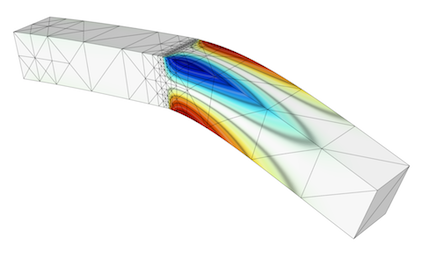This is a version of Example 2 with a simple adaptive mesh refinement loop. The problem being solved is again linear elasticity describing a multi-material cantilever beam. The problem is solved on a sequence of meshes which are locally refined in a conforming (triangles, tetrahedrons) or non-conforming (quadrilaterals, hexahedra) manner according to a simple ZZ error estimator.

The example demonstrates MFEM's capability to work with both conforming and nonconforming refinements, in 2D and 3D, on linear and curved meshes. Interpolation of functions from coarse to fine meshes, as well as persistent GLVis visualization are also illustrated.

The example has a serial (ex21.cpp) and a parallel (ex21p.cpp) version. We recommend viewing Examples 2 and 6 before viewing this example.

## Volta Miniapp: Electrostatics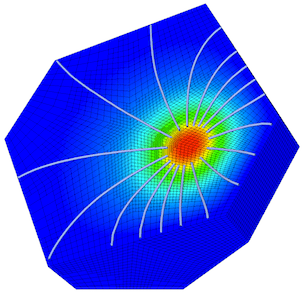This miniapp demonstrates the use of MFEM to solve realistic problems in the field of linear electrostatics. Its features include:

• dielectric materials
• charge densities
• surface charge densities
• prescribed voltages
• applied polarizations
• high order meshes
• high order basis functions

For more details, please see the documentation in the miniapps/electromagnetics directory.

The miniapp has only a parallel (volta.cpp) version. We recommend that new users start with the example codes before moving to the miniapps.

## Tesla Miniapp: Magnetostatics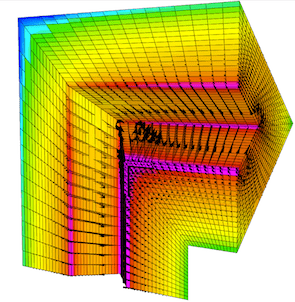This miniapp showcases many of MFEM's features while solving a variety of realistic magnetostatics problems. Its features include:

• diamagnetic and/or paramagnetic materials
• ferromagnetic materials
• volumetric current densities
• surface current densities
• external fields
• high order meshes
• high order basis functions

For more details, please see the documentation in the miniapps/electromagnetics directory.

The miniapp has only a parallel (tesla.cpp) version. We recommend that new users start with the example codes before moving to the miniapps.

## Maxwell Miniapp: Transient Full-Wave Electromagnetics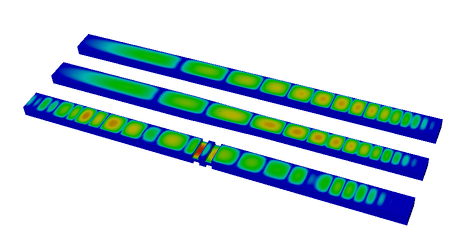This miniapp solves the equations of transient full-wave electromagnetics.

Its features include:

• mixed formulation of the coupled first-order Maxwell equations
• $H(\mathrm{curl})$ discretization of the electric field
• $H(\mathrm{div})$ discretization of the magnetic flux
• energy conserving, variable order, implicit time integration
• dielectric materials
• diamagnetic and/or paramagnetic materials
• conductive materials
• volumetric current densities
• Sommerfeld absorbing boundary conditions
• high order meshes
• high order basis functions

For more details, please see the documentation in the miniapps/electromagnetics directory.

The miniapp has only a parallel (maxwell.cpp) version. We recommend that new users start with the example codes before moving to the miniapps.

## Joule Miniapp: Transient Magnetics and Joule HeatingThis miniapp solves the equations of transient low-frequency (a.k.a. eddy current) electromagnetics, and simultaneously computes transient heat transfer with the heat source given by the electromagnetic Joule heating.

Its features include:

• $H^1$ discretization of the electrostatic potential
• $H(\mathrm{curl})$ discretization of the electric field
• $H(\mathrm{div})$ discretization of the magnetic field
• $H(\mathrm{div})$ discretization of the heat flux
• $L^2$ discretization of the temperature
• implicit transient time integration
• high order meshes
• high order basis functions

For more details, please see the documentation in the miniapps/electromagnetics directory.

The miniapp has only a parallel (joule.cpp) version. We recommend that new users start with the example codes before moving to the miniapps.

## Mobius Strip Miniapp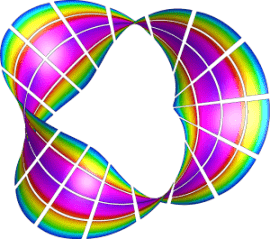This miniapp generates various Mobius strip-like surface meshes. It is a good way to generate complex surface meshes.

Manipulating the mesh topology and performing mesh transformation are demonstrated.

The mobius-strip mesh in the data directory was generated with this miniapp.

For more details, please see the documentation in the miniapps/meshing directory.

The miniapp has only a serial (mobius-strip.cpp) version. We recommend that new users start with the example codes before moving to the miniapps.

## Klein Bottle Miniapp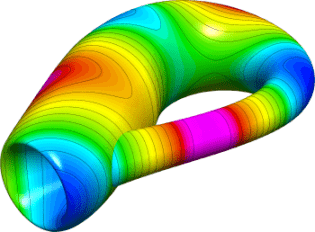This miniapp generates three types of Klein bottle surfaces. It is similar to the mobius-strip miniapp.

Manipulating the mesh topology and performing mesh transformation are demonstrated.

The klein-bottle and klein-donut meshes in the data directory were generated with this miniapp.

For more details, please see the documentation in the miniapps/meshing directory.

The miniapp has only a serial (klein-bottle.cpp) version. We recommend that new users start with the example codes before moving to the miniapps.

## Toroid Miniapp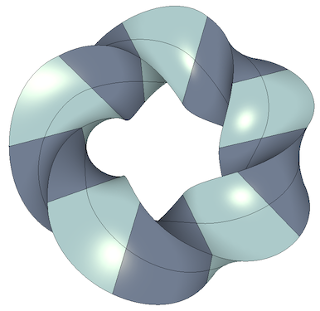This miniapp generates two types of toroidal volume meshes; one with triangular cross sections and one with square cross sections. It works by defining a stack of individual elements and bending them so that the bottom and top of the stack can be joined to form a torus. It supports various options including:

• The element type: 0 - Wedge, 1 - Hexahedron
• The geometric order of the elements
• The major and minor radii
• The number of elements in the azimuthal direction
• The number of nodes to offset by before rejoining the stack
• The initial angle of the cross sectional shape
• The number of uniform refinement steps to apply

Along with producing some visually interesting meshes, this miniapp demonstrates how simple 3D meshes can be constructed and transformed in MFEM. It also produces a family of meshes with simple but non-trivial topology for testing various features in MFEM.

This miniapp has only a serial (toroid.cpp) version. We recommend that new users start with the example codes before moving to the miniapps.

## Extruder Miniapp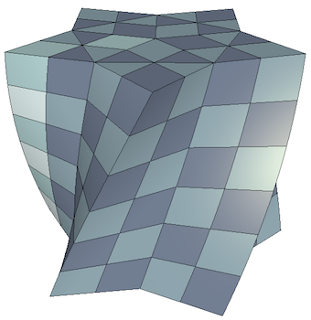This miniapp creates higher dimensional meshes from lower dimensional meshes by extrusion. Simple coordinate transformations can also be applied if desired.

• The initial mesh can be 1D or 2D
• 1D meshes can be extruded in both the y and z directions
• 2D meshes can be triangular, quadrilateral, or contain both element types
• Meshes with high order geometry are supported
• User can specify the number of elements and the distance to extrude
• Geometric order of the transformed mesh can be user selected or automatic

This miniapp provides another demonstration of how simple meshes can be constructed and transformed in MFEM.

This miniapp has only a serial (extruder.cpp) version. We recommend that new users start with the example codes before moving to the miniapps.

## Shaper Miniapp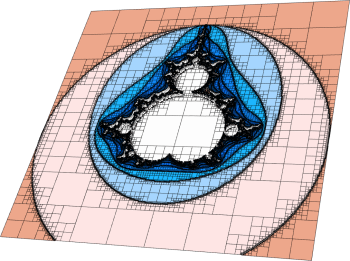This miniapp performs multiple levels of adaptive mesh refinement to resolve the interfaces between different "materials" in the mesh, as specified by a given material function.

It can be used as a simple initial mesh generator, for example in the case when the interface is too complex to describe without local refinement. Both conforming and non-conforming refinements are supported.

For more details, please see the documentation in the miniapps/meshing directory.

The miniapp has only a serial (shaper.cpp) version. We recommend that new users start with the example codes before moving to the miniapps.

## Mesh Explorer Miniapp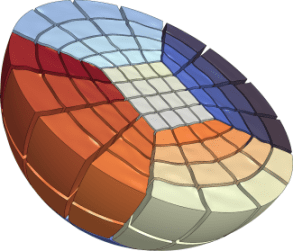This miniapp is a handy tool to examine, visualize and manipulate a given mesh. Some of its features are:

• visualizing of mesh materials and individual mesh elements
• mesh scaling, randomization, and general transformation
• manipulation of the mesh curvature
• the ability to simulate parallel partitioning
• quantitative and visual reports of mesh quality

For more details, please see the documentation in the miniapps/meshing directory.

The miniapp has only a serial (mesh-explorer.cpp) version. We recommend that new users start with the example codes before moving to the miniapps.

## Mesh Optimizer Miniapp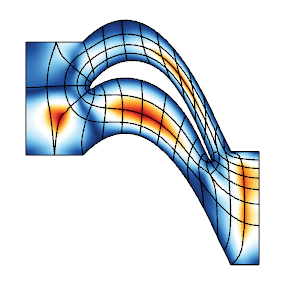This miniapp performs mesh optimization using the Target-Matrix Optimization Paradigm (TMOP) by P.Knupp et al., and a global variational minimization approach. It minimizes the quantity

$$\sum_T \int_T \mu(J(x)),$$

where $T$ are the target (ideal) elements, $J$ is the Jacobian of the transformation from the target to the physical element, and $\mu$ is the mesh quality metric.

This metric can measure shape, size or alignment of the region around each quadrature point. The combination of targets and quality metrics is used to optimize the physical node positions, i.e., they must be as close as possible to the shape / size / alignment of their targets.

This code also demonstrates a possible use of nonlinear operators, as well as their coupling to Newton methods for solving minimization problems. Note that the utilized Newton methods are oriented towards avoiding invalid meshes with negative Jacobian determinants. Each Newton step requires the inversion of a Jacobian matrix, which is done through an inner linear solver.

For more details, please see the documentation in the miniapps/meshing directory.

The miniapp has a serial (mesh-optimizer.cpp) and a parallel (pmesh-optimizer.cpp) version. We recommend that new users start with the example codes before moving to the miniapps.

## Low-Order Refined Transfer Miniapp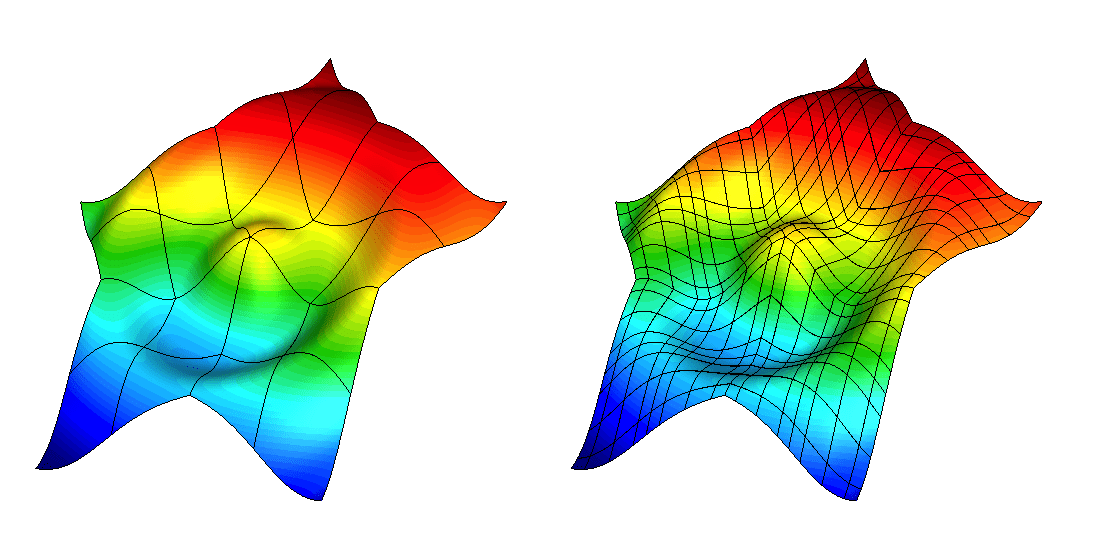The lor-transfer miniapp, found under miniapps/tools demonstrates the capability to generate a low-order refined mesh from a high-order mesh, and to transfer solutions between these meshes.

Grid functions can be transferred between the coarse, high-order mesh and the low-order refined mesh using either $L^2$ projection or pointwise evaluation. These transfer operators can be designed to discretely conserve mass and to recover the original high-order solution when transferring a low-order grid function that was obtained by restricting a high-order grid function to the low-order refined space.

The miniapp has only a serial (lor-transfer.cpp) version. We recommend that new users start with the example codes before moving to the miniapps.

## Laghos Miniapp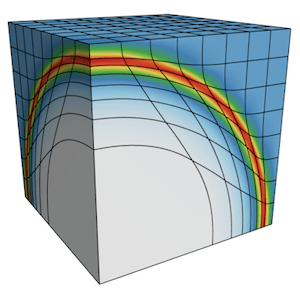Laghos (LAGrangian High-Order Solver) is a miniapp that solves the time-dependent Euler equations of compressible gas dynamics in a moving Lagrangian frame using unstructured high-order finite element spatial discretization and explicit high-order time-stepping.

The computational motives captured in Laghos include:

• Support for unstructured meshes, in 2D and 3D, with quadrilateral and hexahedral elements (triangular and tetrahedral elements can also be used, but with the less efficient full assembly option). Serial and parallel mesh refinement options can be set via a command-line flag.
• Explicit time-stepping loop with a variety of time integrator options. Laghos supports Runge-Kutta ODE solvers of orders 1, 2, 3, 4 and 6.
• Continuous and discontinuous high-order finite element discretization spaces of runtime-specified order.
• Moving (high-order) meshes.
• Separation between the assembly and the quadrature point-based computations.
• Point-wise definition of mesh size, time-step estimate and artificial viscosity coefficient.
• Constant-in-time velocity mass operator that is inverted iteratively on each time step. This is an example of an operator that is prepared once (fully or partially assembled), but is applied many times. The application cost is dominant for this operator.
• Time-dependent force matrix that is prepared every time step (fully or partially assembled) and is applied just twice per "assembly". Both the preparation and the application costs are important for this operator.
• Domain-decomposed MPI parallelism.
• Optional in-situ visualization with GLVis and data output for visualization / data analysis with VisIt.

The Laghos miniapp is part of the CEED software suite, a collection of software benchmarks, miniapps, libraries and APIs for efficient exascale discretizations based on high-order finite element and spectral element methods. See http://github.com/ceed for more information and source code availability.

This is an external miniapp, available at https://github.com/CEED/Laghos.

No examples or miniapps match your criteria.